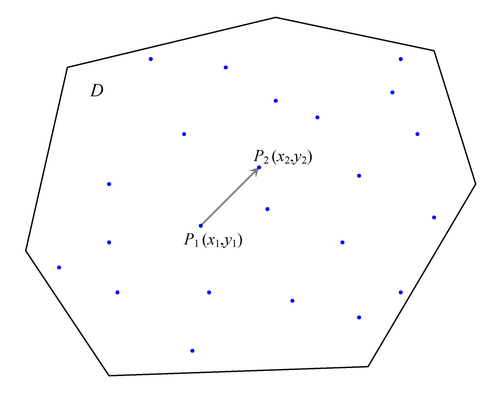#### Pair Formation

The basic starting point in computing the empirical semivariance is the enumeration of pairs of points for the spatial data. Figure 102.19 shows the spatial domain D and the set of n measurements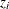,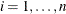, that have been sampled at the indicated locations in D. Two data points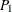and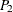, with coordinates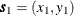and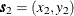, respectively, are selected for illustration.

A vector, or directed line segment, is drawn between these points. If the length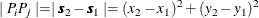of this vector is smaller than the specified DEPSILON= value, then the pair is excluded from the continuity measure calculations because the two pointsandare considered to be at zero distance apart (or collocated). Spatial collocation might appear due to different scales in sampling, observations made at the same spatial location at different time instances, and errors in the data sets. PROC VARIOGRAM excludes such pairs from the pairwise distance and semivariance computations because they can cause numeric problems in spatial analysis.

If this pair is not discarded on the basis of collocation, it is then classified—first by orientation of the directed line segment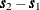, and then by its length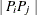. For example, it is unlikely for actual data that the distancebetween any pair of data points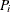and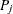located at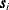and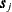, respectively, would exactly satisfy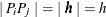in the preceding computation of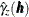. A similar argument can be made for the orientation of the segment. Consequently, the pair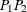is placed into an angle and distance class.

The following subsections give more details about the nature of these classifications. You can also find extensive discussions about the size and the number of classes to consider for the computation of the empirical semivariogram.

Figure 102.19: Selection of Pointsandin Spatial Domain D